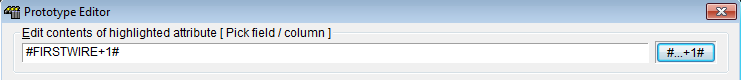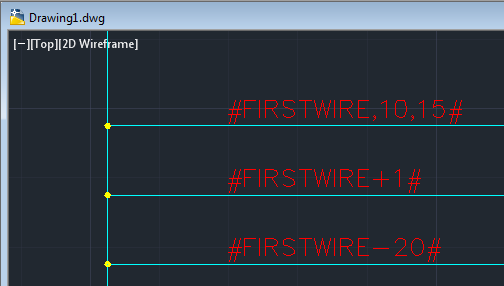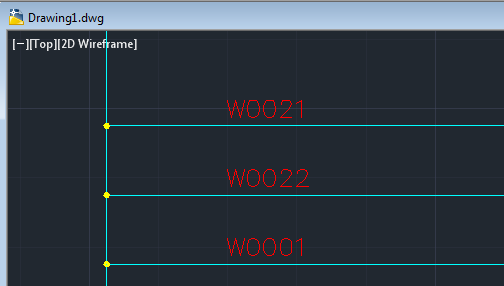# Fields with Mathematical Relationships

## Discussion

It is possible with Protogen to have many text items on a clone drawing linked to the same field in the Protogen database. This will allow you to reduce data entry when the same data is to be shown many times on a single drawing. In Protogen this could be the case for a circuit number or page number or motor/loop number, generally known as a "series number".

It is also possible for alphanumeric data to use the same Protogen field to define many text items that have a simple mathematical relationship, such as a sequence of wire numbers on a drawing; W1, W2, W3. These wires differ only by a mathematical relationship. To encode this type of relationship on to a prototype drawing we use the STEP variation of a field definition.

The STEP field is an optional mathematical relationship to the database field name specified. I.e. If a field name is FIRSTTERM for the first terminal on a circuit and the field definition entered is #FIRSTERM+10# then Protogen will add 10 to the number entered in the database for the field FIRSTERM (e.g. T10) before placing the related number (e.g. T20) in to the drawing. The number can be positive or negative e.g. +1, +50, -20, -1. Leading zeros are preserved when increments or decrements are done. Spaces are NOT ALLOWED between the plus (or minus) sign and the step number!

Within Protoedit, when defining fields, you can press the [+/-] button to set the step number for a field.## Examples• The first wire on a diagram.

#FIRSTWIRE,10,15#. This could be the (normal) definition for the first wire number on a diagram. In the database the column would be 10 characters wide and be the 15th column. An example data entry in this column may be W0021.

• Another wire on the same diagram.

#FIRSTWIRE+1#. This (stepped) field definition could be placed on another wire on the same diagram. This wire would use the same database column as the wire above for its data source. If the data entry in the FIRSTWIRE column was W0021 then this wire would be set to W0022.

• Yet another wire on the same diagram.

#FIRSTWIRE-20#. This (stepped) field definition could be placed on yet another wire on the same diagram. This wire would use the same database column as the first wire above for its data source. If the data entry in the FIRSTWIRE column was W0021 then this wire would be set to W0001.

The result: